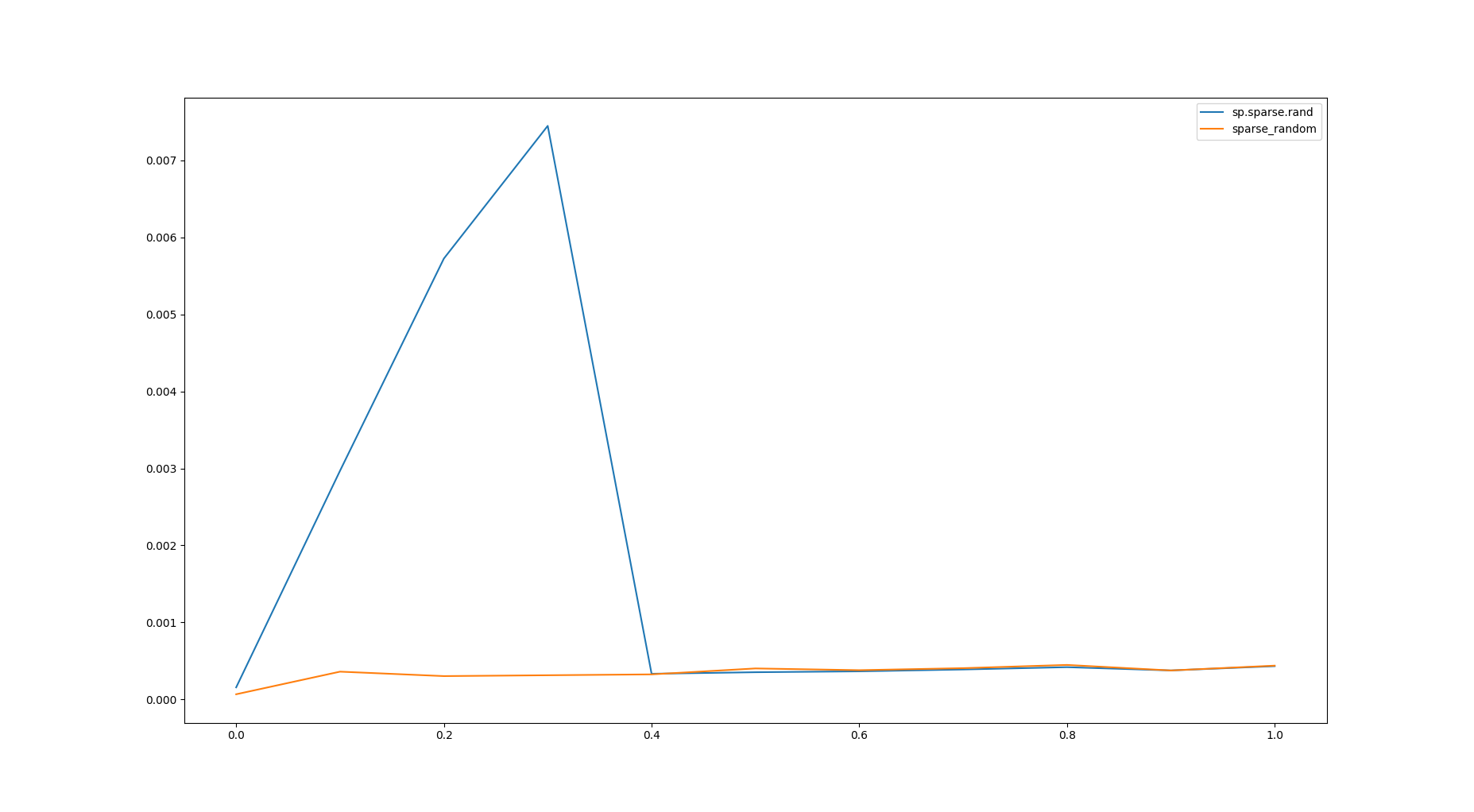# Bizarre times for `scipy.sparse.rand` function with 'low' density values. #9036

Closed
opened this issue Jul 15, 2018 · 2 comments
Closed

# Bizarre times for `scipy.sparse.rand` function with 'low' density values.#9036

opened this issue Jul 15, 2018 · 2 comments
Labels
Milestone

###rwolst commented Jul 15, 2018 • edited

The `scipy.sparse.rand` function is strangely slow for some densities. It seems like it can be made much faster without much effort. The `sparse_random` function below seems to be about 10x faster on the worst case of the `scipy.sparse.rand` function. The timing plots are equally bizarre, showing terrible performance up until around `density=0.4` and then pretty much equivalent timings between both functions.

Judging by the code (https://github.com/scipy/scipy/blob/v1.1.0/scipy/sparse/construct.py#L775), it looks to be caused by this conditional

``````    # Use the algorithm from python's random.sample for k < mn/3.
if mn < 3*k:
ind = random_state.choice(mn, size=k, replace=False)
else:
ind = np.empty(k, dtype=tp)
selected = set()
for i in xrange(k):
j = random_state.randint(mn)
while j in selected:
j = random_state.randint(mn)
``````

I'm not sure why this is even there and it is not simply

``````ind = random_state.choice(mn, size=k, replace=False)
``````

### Reproducing code example:

``````import scipy as sp
import scipy.sparse
import numpy as np
from contexttimer import Timer
import matplotlib.pyplot as plt

def sparse_random(n_rows, n_cols, density):
"""A faster implementation of scipy.sparse.rand?"""
if density == 0:
return sp.sparse.coo_matrix((n_rows, n_cols))
N = n_rows*n_cols
nnz = int(N*density)
idx = np.random.choice(N, nnz, replace=False)
rows, cols = np.divmod(idx, n_cols)
data = np.random.rand(nnz)
return sp.sparse.coo_matrix((data, (rows, cols)), shape=(n_rows,n_cols))

def time_test(n_rows, n_cols):
densities = np.arange(0, 1.1, 0.1)
times_sp = np.empty(densities.size)
times = np.empty(densities.size)
for i, density in enumerate(densities):
with Timer() as t:
sp.sparse.rand(n_rows,n_cols,density)
times_sp[i] = t.elapsed

with Timer() as t:
sparse_random(n_rows,n_cols,density)
times[i] = t.elapsed
plt.plot(densities, times_sp)
plt.plot(densities, times)
plt.legend(['sp.sparse.rand', 'sparse_random'])
plt.show()

time_test(120, 80)
``````

My timings are below:### Scipy/Numpy/Python version information:

``````In : import sys, scipy, numpy; print(scipy.__version__, numpy.__version__, sys.version_info)
1.1.0 1.14.5 sys.version_info(major=3, minor=6, micro=4, releaselevel='final', serial=0)
``````

###perimosocordiae commented Jul 16, 2018

 It turns out this is mostly a historical artifact. Here's the commit that added the code in question: 589c372 We couldn't use `random_state.choice` until numpy 1.7 became the minimum required version. The workaround using `random_state.permutation` was inefficient for small densities, so a special case was added. When the numpy version bump happened in commit aa6bc5b, we didn't notice that the special case was no longer needed (and apparently slower, as well). Feel free to send a PR with the fix, if you'd like!

###rwolst commented Jul 17, 2018

 Ok, I'll fix this.
added a commit to rwolst/scipy that referenced this issue Jul 17, 2018
``` Fix slow sparse.rand for k < mn/3 (scipy#9036). ```
``` fda2bca ```
added a commit to rwolst/scipy that referenced this issue Aug 31, 2018
``` MAINT: fix slow sparse.rand for k < mn/3 (scipygh-9036). ```
``` 895e3cf ```
added a commit to rwolst/scipy that referenced this issue Nov 7, 2018
``` MAINT: fix slow sparse.rand for k < mn/3 (scipygh-9036). ```
``` 666f8b0 ```
added a commit that referenced this issue Nov 7, 2018
``` Merge pull request #9051 from rwolst/sparse_rand_slow_fixe ```
``` 93fe083 ```
`MAINT: Fix slow sparse.rand for k < mn/3 (#9036).`